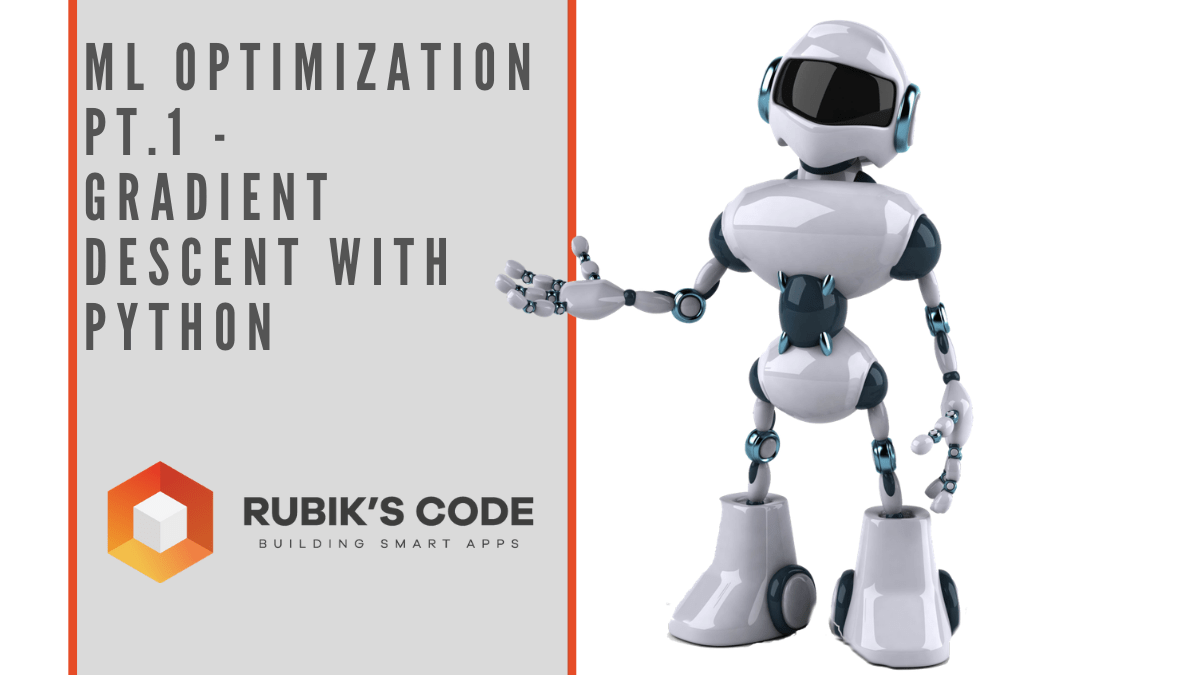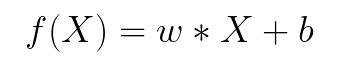# ML Optimization pt.1 - Gradient Descent with PythonIn this article, we explore gradient descent - the grandfather of all optimization techniques and it’s variations. We implement them from scratch with Python.

So far in our journey through the Machine Learning universe, we covered several big topics. We investigated some regression algorithms, classification algorithms and algorithms that can be used for both types of problems (SVM, **[Decision Trees](https://rubikscode.net/2020/09/28/back-to-machine-learning-basics-decision-tree-random-forest/) and Random Forest). Apart from that, we dipped our toes in unsupervised learning, saw how we can use this type of learning for [clustering**](https://rubikscode.net/2020/10/05/back-to-machine-learning-basics-clustering/) and learned about several clustering techniques.

We also talked about how to quantify machine learning model performance and how to improve it with regularization. In all these articles, we used Python for “from the scratch” implementations and libraries like TensorFlowPytorch and SciKit Learn. The word optimization popped out more than once in these articles, so in this and next article, we focus on optimization techniques which are an important part of the machine learning process.

In general, every machine learning algorithm is composed of three integral parts:

1. loss function.
2. Optimization criteria based on the loss function, like a cost function.
3. Optimization technique – this process leverages training data to find a solution for optimization criteria (cost function).

As you were able to see in previous articles, some algorithms were created intuitively and didn’t have optimization criteria in mind. In fact, mathematical explanations of why and how these algorithms work were done later. Some of these algorithms are Decision Trees and kNN. Other algorithms, which were developed later had this thing in mind beforehand. SVMis one example.

During the training, we change the parameters of our machine learning model to try and minimize the loss function. However, the question of how do you change those parameters arises. Also, by how much should we change them during training and when. To answer all these questions we use optimizers. They put all different parts of the machine learning algorithm together. So far we mentioned Gradient Decent as an optimization technique, but we haven’t explored it in more detail. In this article, we focus on that and we cover the grandfather of all optimization techniques and its variation. Note that these techniques are not machine learning algorithms. They are solvers of minimization problems in which the function to minimize has a gradient in most points of its domain.

### Dataset & Prerequisites

Data that we use in this article is the famous Boston Housing Dataset . This dataset is composed 14 features and contains information collected by the U.S Census Service concerning housing in the area of Boston Mass. It is a small dataset  with only 506 samples.For the purpose of this article, make sure that you have installed the following _Python _libraries:

Once installed make sure that you have imported all the necessary modules that are used in this tutorial.

``````import pandas as pd
import numpy as np
import matplotlib.pyplot as plt

from sklearn.model_selection import train_test_split
from sklearn.metrics import mean_squared_error
from sklearn.preprocessing import StandardScaler
from sklearn.linear_model import SGDRegressor``````

Apart from that, it would be good to be at least familiar with the basics of linear algebracalculus and probability.

### Why do we use Optimizers?

Note that we also use simple Linear Regression in all examples. Due to the fact that we explore optimizationtechniques, we picked the easiest machine learning algorithm. You can see more details about Linear regression here. As a quick reminder the formula for linear regression goes like this:where w and b are parameters of the machine learning algorithm. The entire point of the training process is to set the correct values to the w and b, so we get the desired output from the machine learning model. This means that we are trying to make the value of our error vector as small as possible, i.e. to find a global minimum of the cost function.

One way of solving this problem is to use calculus. We could compute derivatives and then use them to find places where is an extrema of the cost function. However, the cost function is not a function of one or a few variables; it is a function of all parameters of a machine learning algorithm, so these calculations will quickly grow into a monster. That is why we use these optimizers.

## AI vs ML vs DL What is Artificial Intelligence Machine Learning Deep Learning? General vs Narrow AI

What is the difference between AI ML and DL. What is ANI vs AGI. When will general artificial intelligence be a reality? What can narrow artificial intelligence do today that is better than human intelligence?

## Applied Data Analysis in Python Machine Learning and Data Science | Scikit-Learn

Applied Data Analysis in Python Machine learning and Data science, we will investigate the use of scikit-learn for machine learning to discover things about whatever data may come across your desk.

## Back to Machine Learning Basics - Support Vector Machines

In this article we explore SVM for regression and classification, implement it from scratch with Python and learn how to use SciKit Learn implementation.

## PyTorch for Deep Learning | Data Science | Machine Learning | Python

PyTorch is a library in Python which provides tools to build deep learning models. What python does for programming PyTorch does for deep learning.

## Data Science Projects | Data Science | Machine Learning | Python

Practice your skills in Data Science with Python, by learning and then trying all these hands-on, interactive projects, that I have posted for you.